/
/
/
211) Find the derivative of the function f(x, y, z)
Not my Question
Flag Content

# Question : 211) Find the derivative of the function f(x, y, z) : 1757202

211) Find the derivative of the function f(x, y, z) = ln(xy + yz + zx) at the point (-9, -18, -27) in the direction in which the function decreases most rapidly.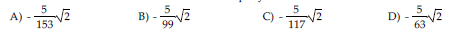212) Find the derivative of the function f(x, y, z) = x/y + y/z + z/x at the point (9, -9, 9) in the direction in which the function increases most rapidly.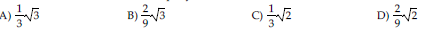213) Find the derivative of the function f(x, y, z) = x/y + y/z + z/x at the point (-10, 10, -10) in the direction in which the function decreases most rapidly.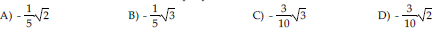214) Write an equation for the tangent line to the curve x2/64 + y2/ 16 = 1 at the point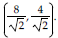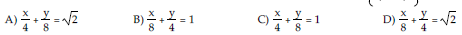215) Write an equation for the tangent line to the curve xy = 20 at the point (5, 4).

A) 5x + 4y = 40 B) 4x + 5y = 40 C) 4x + 5y = 20 D) 5x + 4y = 20

216) Write an equation for the tangent line to the curve x2 - 4xy + y2 = 6 at the point (-1, 1).

A) x - y + 2 = 0 B) y = x + 1 C) x + y = 1 D) y = x - 2

217) Write an equation for the tangent line to the curve y2 - x = 4 at the point (1, 5).

A) x - 2y + 1 = 0 B) x - 2y + 9 = 0

C) x - 2 5y + 1 = 0 D) x - 2 5y + 9 = 0

218) Find the equation for the tangent plane to the surface 5x + 4y + 9z = 19 at the point (1, -1, 2).

A) 5x + 4y + 9z = 18 B) 5x - 4y + 18z = 19

C) 5x + 4y + 9z = 19 D) 5x - 4y + 18z = 18

219) Find the equation for the tangent plane to the surface z = -8x2 - 3y2 at the point (2, 1, -35).

A) -32x - 6y - z = -35 B) 2x + y - 35z = 1

C) 2x + y - 35z = -32 D) -32x - 6y - z = -40

220) Find the equation for the tangent plane to the surface x2 + 7xyz + y2 = -5z2 at the point (-1, -1, -1).

A) x + y + z = 1 B) x + y + z = -7

C) 5x + 5y - 3z = -7 D) 5x + 5y - 3z = 1

## Solution 5 (1 Ratings )

Solved
Mathematics 3 Years Ago 60 Views